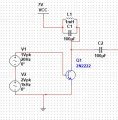# Derivation of mathematical expression for AM-signal.

Thread Starter

#### JohanEricson

Joined Aug 30, 2017
32
So I've been looking around the internet for a good explanation/derivation of the expression e = (Ec+Ei*sin(wi*t)}*sin(wc*t)
Through this expression, it's very clear that the amplitude of the carrier wave is time-varying with the frequency of the intelligence wave.

But the question is how do we get here?
I've seen loads of derivations where they partially show how it's done, like:
e = A*sin(wc*t)
A = Ec+Ei*sin(wi*t)
---> e = (Ec+Ei*sin(wi*t))*sin(wc*t)

But this still assumes that e = A*sin(wc*t) is known.

I've read on the Swedish version of Wikipedia that "the nonlinear device" (which is not very well explained by nor the book or our teacher.) is supposed to be as quadratic as possible.

Any way... I simulated this circuit, and it produced the expected result, an amplitude modulated signal.The signal going into the base of the transistor is the intelligence added to the carrier, Ec+Ei.

So what is happening in the "non-linear device" to go from
Ec*sin(wc*t) + Ei*sin(wi*t) ----non lienar---> e = (Ec+Ei*sin(wi*t)}*sin(wc*t)

I've also read about the upper and lower sideband has something to do with the result, and that these side frequencies come as a result of the non-linear device.

So if someone could provide a bit of insight into this matter would be great!
I realize that it's probably much more complicated than I can imagine.

Thank you!

Last edited:

#### danadak

Joined Mar 10, 2018
4,057
Last edited:

#### MrAl

Joined Jun 17, 2014
8,266
So I've been looking around the internet for a good explanation/derivation of the expression e = (Ec+Ei*sin(wi*t)}*sin(wc*t)
Through this expression, it's very clear that the amplitude of the carrier wave is time-varying with the frequency of the intelligence wave.

But the question is how do we get here?
I've seen loads of derivations where they partially show how it's done, like:
e = A*sin(wc*t)
A = Ec+Ei*sin(wi*t)
---> e = (Ec+Ei*sin(wi*t))*sin(wc*t)

But this still assumes that e = A*sin(wc*t) is known.

I've read on the Swedish version of Wikipedia that "the nonlinear device" (which is not very well explained by nor the book or our teacher.) is supposed to be as quadratic as possible.

Any way... I simulated this circuit, and it produced the expected result, an amplitude modulated signal.
View attachment 150252
The signal going into the base of the transistor is the intelligence added to the carrier, Ec+Ei.

So what is happening in the "non-linear device" to go from
Ec*sin(wc*t) + Ei*sin(wi*t) ----non lienar---> e = (Ec+Ei*sin(wi*t)}*sin(wc*t)

I've also read about the upper and lower sideband has something to do with the result, and that these side frequencies come as a result of the non-linear device.

So if someone could provide a bit of insight into this matter would be great!
I realize that it's probably much more complicated than I can imagine.

Thank you!
Hello,

In the basic theory you consider the "nonlinear device" to be a simple multiplier. That shows the basic theory behind AM. The carrier is modulated by the offset message signal so that the carrier amplitude varies as the message signal varies and thus the changes in carrier amplitude can be detected at a distance with an appropriate receiver. It is actually very simple in theory because it is the multiplication of two waves, that's all.

If we have a message signal M then:
F1=1+M*cos(wm*t)

and carrier:
F2=A*sin(wc*t)

and the result is:
F3=F1*F2=sin(t*wc)*A*(cos(t*wm)*M+1)

and using trig identities we get:
F3=(sin(t*wm+t*wc)*A*M)/2-(sin(t*wm-t*wc)*A*M)/2+sin(t*wc)*A

and you'll notice we get half the sum and difference frequencies wm and wc plus the carrier.
(A*M*(sin(t*(wm+wc))-A*M*sin(t*(wm-wc)))/2+A*sin(t*wc))

and then you can notice that we see A*M in front of the first two terms which shows the multiplication of the two amplitudes, with the carrier added.

If it is the implementation you are after, there are many ways to get this result. The simpliest conceptually is of course to use a multiplier circuit#### crutschow

Joined Mar 14, 2008
27,243
I've also read about the upper and lower sideband has something to do with the result, and that these side frequencies come as a result of the non-linear device.
and you'll notice we get half the sum and difference frequencies wm and wc plus the carrier.
(A*M*(sin(t*(wm+wc))-A*M*sin(t*(wm-wc)))/2+A*sin(t*wc))
In case it's not clear, the sum and difference frequencies are the upper and lower sidebands that are produced by this multiplication of the two sine-waves.

Similar threads